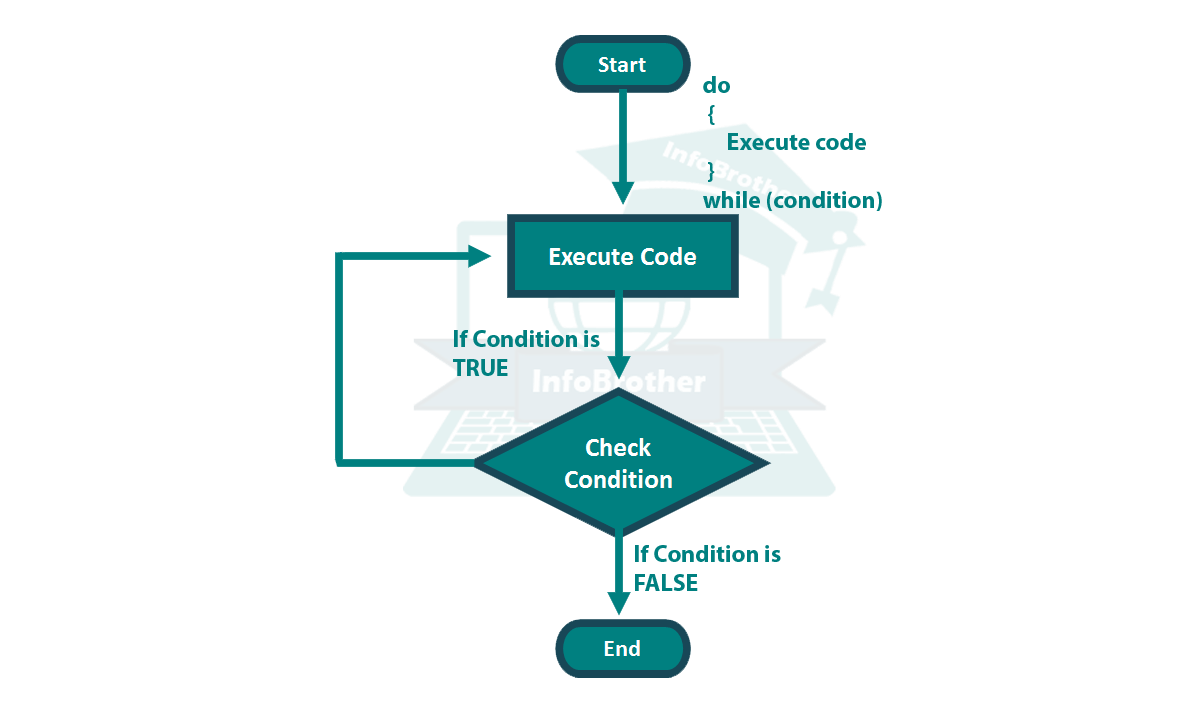Topic : Do-while Loop in C++ Programming Language:

### Do-while Loop:

Do-while Loop is same like while loop, Only the difference is that In do-while Loop, the statement will execute at least once. even if the given condition is False. Do-while Loop execute the statement first, and then check the condition. if the condition is true, will execute the statement second time, otherwise Program will be over as shown in figure.### Syntax:

The Syntax of the do-while Loop is:

``` do { //do-block containing one or more C++ statements. }   while ( condition ); ```

The condition is tested at the end of the loop body instead of the beginning; therefore, the body of the loop will be executed at least once before the condition is checked. If the condition is true, we jump back to the beginning of the block and execute it again. A do-while loop is basically a reversed while loop. A while loop says, "Loop while the condition is true, and execute this block of code", a do-while loop says, "Execute this block of code, and then loop back while the condition is true".

Note that there is an semicolon (;) after the close parentheses. and there is no block for "while" here.

Example:

Let's have a simple example to understand how do-while-loop actually work. In this example, we will create a simple Game. we will hide a number here and can ask our friend to Guess the number. This loop will be executed repeatedly until your friend Guess the right Number.

``` /* Guessing Game: InfoBrother */   #include <iostream> using namespace std; main() { int number=55; /*Need to be guess this Number:*/ int guess; /*User guess will store here.*/   cout<<" Guess Number: "; cin>>guess;     /*while guess is not equal to 55, execute loop-body again and again. until loop-condition become false. mean Guess become equal to 55*/     while (guess != number) { //body begin. /*the body of while-loop will execute until user Guess right number.*/ cout<<" Wrong Guess...!!" <<endl; cout<<" \nGuess again: "; cin>>guess; } //body close.     /*if user Guess right Number, then the condition of while-loop become false and the loop will be over and the next statement will execute.*/   cout<<" Congrats this is the right Guess. :"<<endl; return 0; } ```

Example:

Let's have another Useful Example, which will show some real meaning of do-while Loop. in this example, we will make a small calculator. this calculator will ask you to enter numbers. this statement will execute again and again until you entered zero (0). when you will enter zero, the while condition will become false and a loop will terminate. after termination the Loop, it will show you the sum of all entered Numbers.

``` /*Simple calculator using do-While Loop*/ #include<iostream> using namespace std;   main() { int number=0; //will used to store Numbers from user. int sum=0; //will used to store sum of all entered Numbers.   /*This loop will ask you to enter number again and again until while condition became false.*/ do { cout<<"Please Enter The Number To Add: "; cin>>number; sum= sum+number; //will add entered numbers and store in "Sum". }   //execution of do-block will continue until you enterd zero (0). while(number!=0); //when the while condition become False.this statement will execute. cout<<"\n\nThe Sum of All Entered Numbers are: "<<sum;   return 0; } ```

I Tried my Best to Provide you complete Information regarding this topic in very easy and conceptual way. but still if you have any Problem to understand this topic, or do you have any Questions, Feel Free to Ask Question. i'll do my best to Provide you what you need.

Sardar Omar.
InfoBrother